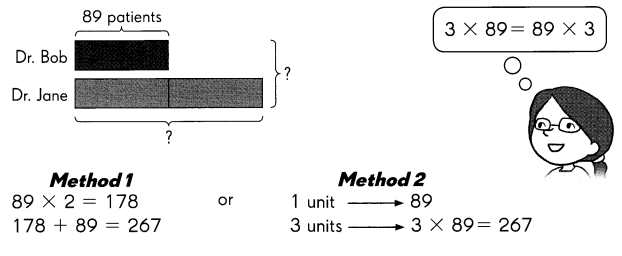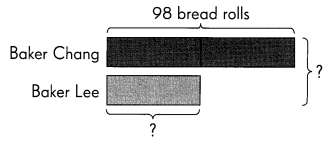# Math in Focus Grade 3 Chapter 9 Answer Key Using Bar Models: Multiplication and Division

Practice the problems of Math in Focus Grade 3 Workbook Answer Key Chapter 9 Using Bar Models: Multiplication and Division to score better marks in the exam.

## Math in Focus Grade 3 Chapter 9 Answer Key Using Bar Models: Multiplication and Division

Math Journal

Look at the bar models, number sentences, and the answer statement. Write a question that matches this problem.

Question 1.Both doctors see 267 patients.

My question:
Dr. Bob see 89 patients daily. Dr. Jane sees patients  twice of Dr. Bob. So How many patients will see daily Dr. Bob and Dr. Jane altogether?

Look at the bar models and the answer statement. Write a question that matches this problem and show two possible methods tor solving the word problem.

Question 2.Both bakers bake 147 bread rolls.

My question:
Baker Chang  bake 98 bread rolls.. Baker Chang  baked 2 times as many bread rolls as Baker Lee. How many bread rolls did Baker Lee?

My methods:
Method 1:
98  ÷  2= 49
Method 2:
3 units –> 147
1 unit –> 147 ÷ 3 = 49

Challenging Practice

Timmy and Pedro have 24 trading cards altogether. Timmy gives Pedro 5 cards, and they each have the same number of cards. How many trading cards does Pedro have at first?
7 trading cards Pedro have at first
Explanation:
24 ÷ 2 = 12
if both has same number of cards, then each has 12 cards.
Timmy gives Pedro 5 cards, and they each have the same number of cards.
So Pedro has earlier is 12 – 5  =7

Problem Solving